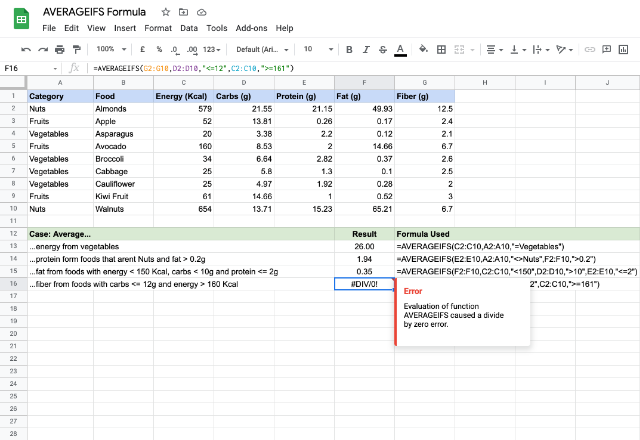# How to use the AVERAGEIFS Google Sheets formulaThe AVERAGEIFS Google Sheets formula is similar to that of the AVERAGEIF Google Sheets formula, but with a slight difference. While the AVERAGEIF returns the average of values satisfying a single criteria, the AVERAGEIFS formula gives us the average of values subject to multiple criteria.

### Syntax

AVERAGEIFS(average_range, criteria_range1, criterion1, [criteria_range2, criterion2, …])

• average_range – is the address reference to the range of cells that the AVERAGEIFS formula considers for averaging. Unlike the AVERAGEIF formula, this is the first parameter within this formula.
• criteria_range1 – this is the range of cells against which the formula checks for the criterion1.
• criterion1 – is the condition or test that is used to qualify cells within the criteria_range1 for averaging. There are six types of comparisons in general, as listed below. While we can compare both numbers and text using the first two operators, we can only compare numbers using the last four operators.
• Equals (=)
• Not equal to (<>)
• Greater than (>)
• Greater than or equal to (>=)
• Less than (<)
• Less than or equal to (<=)
• criteria_range2, criterion2, … – these are optional and additional ranges and criteria that the AVERAGEIFS formula checks for.

### Usage: AVERAGEIFS Google Sheets formula

Below is a sample nutritional information from a select set of foods. Let us try a few examples to establish our understanding of the formula further.We have the sample data populated on the cells A1 through to G10. Please notice the first case has a single criterion, the second one has two and third has three.

Please consider the second case in row # 14. Using the parameters we’ve entered, the AVERAGEIFS formula checks for instances of anything not named “Nuts” within the range A2:A10, then checks for occurrences where values in F2:F10 are greater than 0.2, and finally averages the values from the range E2:E10 that are in the rows that qualify the above two conditions.

Now, let us spend a moment on the case in row # 16. Interestingly, the formula returned an error here because it did not find any matching rows for the criteria specified. Zero instance matching implies a divide by zero instance, hence the error.

### AVERAGEIFS formula

And there you go! Use the AVERAGEIFS formula in Google Sheets to return the average of values subject to multiple criteria.

If you’d like to learn more about the various formulas of Google Sheets, why not take a look at our blog post on the DAVERAGE formula in Google Sheets.

Alternatively, check out related blog posts below!